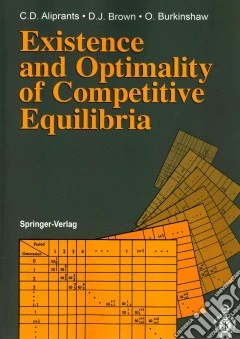ricerca
avanzata

# Existence and Optimality of Competitive Equilibria

## Un libro in lingua di Aliprantis Charalambos D.Brown Donald J.Owen Burkinshaw edito da Springer Verlag, 1990

• € 94,90
• Il prezzo è variabile in funzione del cambio della valuta d’origineThis book presents the theory of general economic equilibrium from a modern perspective. It gives a systematic exposition of research done by the authors and others on the subject of general equilibrium theory over the last ten years. It is intended to serve both as a graduate text on aspects of general equilibrium theory and as an introduction, for economists and mathematicians working in mathematical economics, to current research in a frontier area of general equilibrium theory. To make the material as accessible as possible to the student, the authors have provided two introductory chapters on the basic Arrow-Debreu economics model and the mathematical framework. Exercises at the end of each section complement the exposition. The monograph addresses the questions of existence and optimality of Walrasian equilibria for economies with a finite number of households and firms, but with an infinite number of commodities. The final chapter of the book presents a comprehensive study of the overlapping generations model. This is the first book to give a unified and mathematically rigorous presentation of the theory of general economic equilibrium in an infinite dimensional setting.